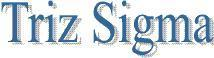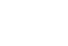Regression Introduction Regression analysis is the statistical technique that identifies the relationship between two or more quantitative variables: a dependent variable whose value is to be predicted, and an independent or explanatory variable (or variables), about which knowledge is available. The technique is used to find the equation that represents the relationship between the variables. A statistical measurement of correlation can be calculated using the least squares method to quantify the strength of the relationship between two variables. The output of that calculation is the Correlation Coefficient, or (r), which ranges between -1 and 1. A value of 1 indicates perfect positive correlation - as one variable increases, the second increases in a linear fashion. Likewise, a value of -1 indicates perfect negative correlation - as one variable increases, the second decreases. A value of zero indicates zero correlation. Before calculating the Correlation Coefficient, the first step is to construct a scatter diagram. Most spreadsheets, including Excel, can handle this task. Looking at the scatter diagram will give you a broad understanding of the correlation. Simple Regresion Analysis Independent Variables are characteristics that can be measured directly (example the area of a house). These variables are also caled predictor variables (used to predict the dependent variable) or explanatory variables (used to explain the behavior of the dependent variable). Dependent variable is a characteristic whose value depends on the values of independent variables. Y (Dependent Variable) = B0 (Constant Term - Intercept) + B1 (Slope coefficient)* X 1 (Independent Variable) + E (error / Regidual) Here is an example of Simple Linear Regression.Y=1636.415+1.487X The slope of 1.487 means that for each increase of one unit in X, we predict the average of Y to increase by an estimated 1.487 units. The equation estimates that for each increase of 1 square foot in the size of the store, the expected annual sales are predicted to increase by \$1487. Calculations - Example 2 Here we give one independent variable (X) and one dependent variable (Y)But how much of the variance in Y (and thus in SST) can be explained by changes in the values of X (SSR), and how much is just due to random error (SSE)? SST (Total Sample Variability,Total Sum of Squares ) = SSR (Explained Variability,Regression Sum of Squares ) + SSE (Unexpained Variability, Error Sum of Squares)Coefficient of Determination (r2) = SSR/SST= 183.333/187.333 = .9786 0< r2 <1; the closer to 1, the better the fit Correlation Coefficient r = (sign of b1)* Sqrt( r2) =+0.9892